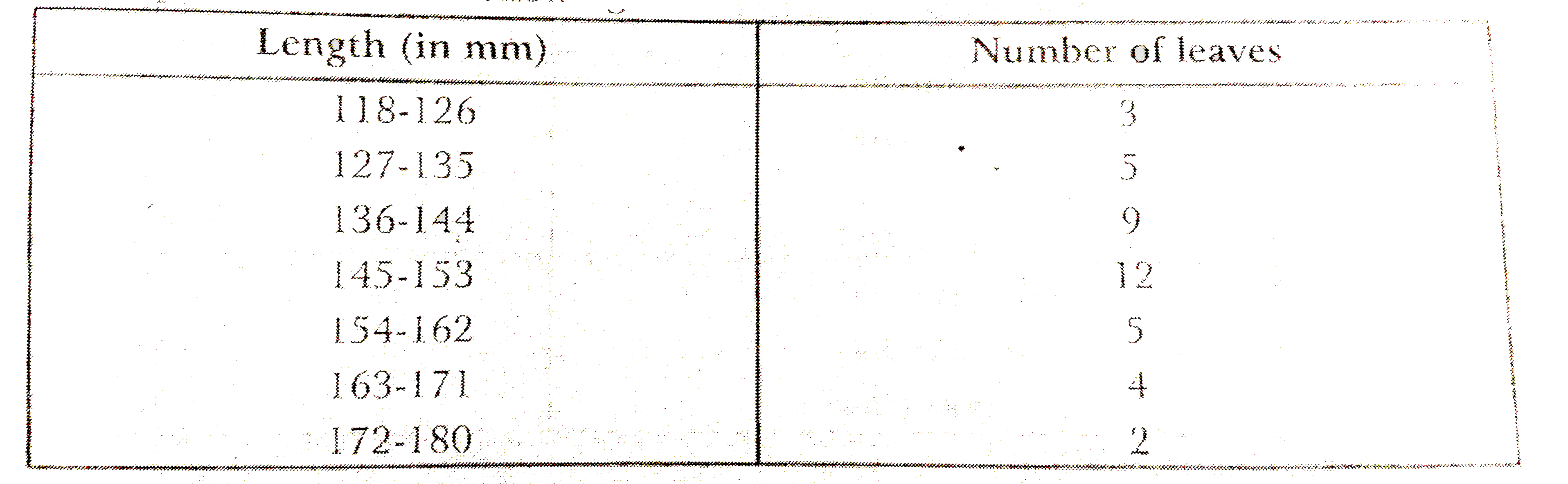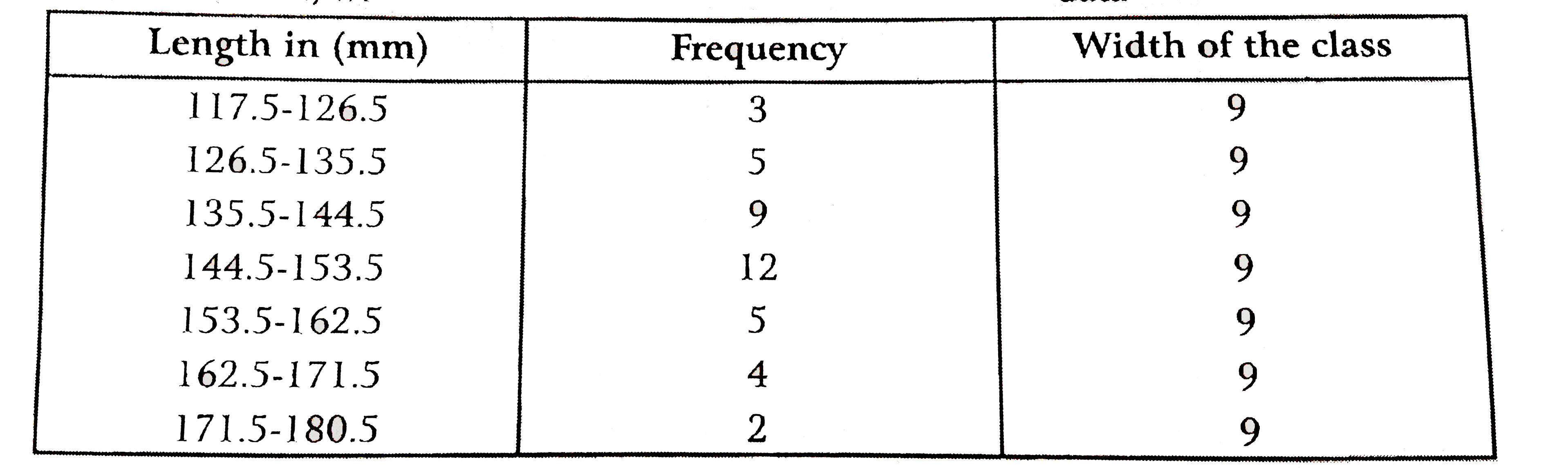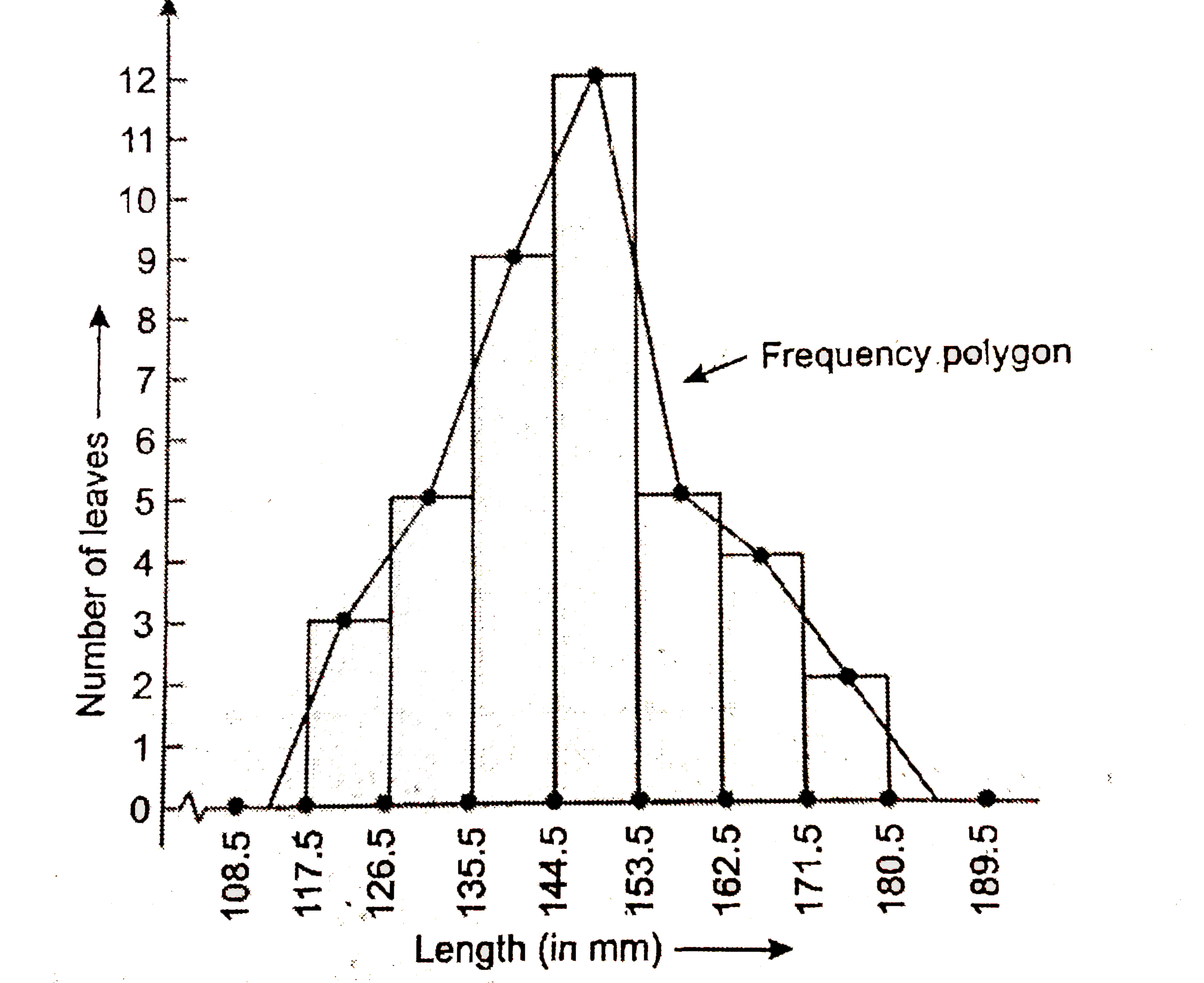# The length of 40 leaves of a plant measured correct to one millimetre and the obtained data is represented in the following table: (i) Draw a histogra

53 views

closed
The length of 40 leaves of a plant measured correct to one millimetre and the obtained data is represented in the following table:(i) Draw a histogram to represent the given data.
(ii) Is there any other suitable graphical representation for the same data?
(iii) Is it correct to conclude that the maximum number of leaves 153 mm long and why?

by (97.1k points)
selected by

(i) We know that, the areas of the rectangles are proportional to the frequencies in a histogram. Now, we get the following modified table by given dataNow, we draw the histogram for given data:(ii) Frequency polygon.
(iii) No, because the maximum number of leaves have their lengths lying in the whole interval 145-153.